# Predict Stocks using Monte Carlo Simulation¶In this notebook we will be looking at data from the stock market, particularly some technology stocks, otherwise known as FAANG stocks. We will learn how to use pandas to get stock information, visualize different aspects of it, and finally we will look at a few ways of analyzing the risk, return, based on its previous performance history. We will also be predicting future stock prices through a Monte Carlo Simulation. Along the way we'll be asking the following questions:

• What was the change in price of the stock over time?
• What was the daily return of the stock on average?
• What was the daily/annual risk of the stocks in the portfolio?
• What was the correlation between different stocks'?
• How much value do we put at risk by investing in a particular stock?
• How can we attempt to predict future stock behavior? (Predicting the closing stock price using Monte Carlo Simulation)
In :
# Importing relevant libraries
import yfinance as yf
import numpy as np
import pandas as pd
import seaborn as sns
from pandas_datareader import data as pdr
import matplotlib.pyplot as plt

In :
# Importing finance data from YFinance API
yf.pdr_override()
tickers = ['FB', 'AAPL', 'AMZN', 'NFLX', 'GOOG']
start_date = '2017-4-1'
end_date = '2022-4-4'

[*********************100%***********************]  5 of 5 completed

In :
port.head()

Out:
AAPL AMZN FB GOOG NFLX
Date
2017-03-31 33.859760 44.327000 142.050003 41.478001 147.809998
2017-04-03 33.869179 44.575500 142.279999 41.927502 146.919998
2017-04-04 34.121380 45.341499 141.729996 41.728500 145.500000
2017-04-05 33.944611 45.464001 141.850006 41.570499 143.619995
2017-04-06 33.859760 44.914001 141.169998 41.394001 143.740005
In :
port.describe()

Out:
AAPL AMZN FB GOOG NFLX
count 1261.000000 1261.000000 1261.000000 1261.000000 1261.000000
mean 80.123107 109.871128 218.853751 76.887053 377.382165
std 44.705114 42.834616 64.826336 31.930668 132.209092
min 33.157391 44.233501 124.059998 41.167500 139.759995
25% 42.285892 80.153503 171.470001 54.124001 291.559998
50% 55.077782 94.214996 191.550003 61.496498 361.410004
75% 122.838203 157.935501 266.720001 89.088501 494.250000
max 181.511703 186.570496 382.179993 150.709000 691.690002

# What was the change in price of the stock over time?¶

In :
#Normalize the data to 100 and plot the historial price on a graph.
(port / port.iloc * 100).plot(figsize=(15, 6));
plt.xlabel('Time(Years)')
plt.ylabel('Price($)') plt.show()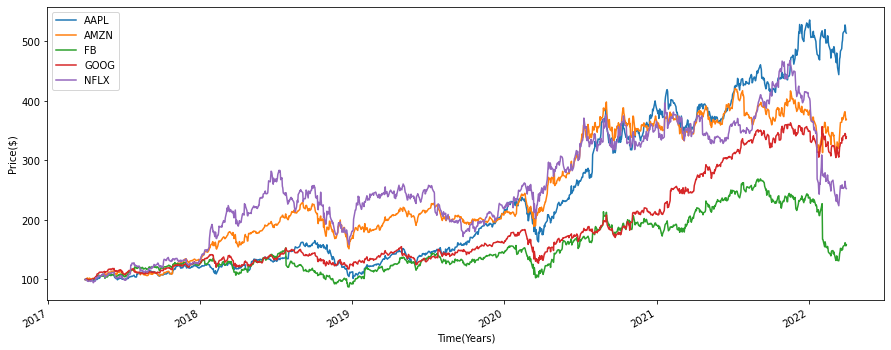The graph above gives us a general overiew of the prices of the stocks in our portfolio overtime. We can see that over the 5 year time period we've selected, AAPL has generated the highest overall return whilst FBappears to have generated the lowest return in the same time period with AMZN, NFLX and GOOG generating returns in between. In : from plotly.subplots import make_subplots import plotly.graph_objects as go # Initialize figure with subplots fig = make_subplots( rows=5, cols=1, subplot_titles=("Apple Stock Price", "Amazon Stock Price", "Facebook Stock Price", "Google Stock Price", 'Netflix') ) # Add traces fig.add_trace(go.Scatter(name='Apple', x=(port.index), y=port['AAPL']), row=1, col=1) fig.add_trace(go.Scatter(name='Amazon', x=port.index, y=port['AMZN']), row=2, col=1) fig.add_trace(go.Scatter(name='Facebook',x=port.index, y=port['FB']), row=3, col=1) fig.add_trace(go.Scatter(name='Google', x=port.index, y=port['GOOG']), row=4, col=1) fig.add_trace(go.Scatter(name='Netflix' ,x=port.index, y=port['NFLX']), row=5, col=1) # Update xaxis properties fig.update_xaxes(title_text="Time(Years)", showgrid=False,row=1, col=1) fig.update_xaxes(title_text="Time(Years)", showgrid=False,row=2, col=1) fig.update_xaxes(title_text="Time(Years)", showgrid=False, row=3, col=1) fig.update_xaxes(title_text="Time(Years)", showgrid=False, row=4, col=1) fig.update_xaxes(title_text="Time(Years)", showgrid=False, row=5, col=1) # Update yaxis properties fig.update_yaxes(title_text="Price($)", row=1, col=1)
fig.update_yaxes(title_text="Price($)", row=2, col=1) fig.update_yaxes(title_text="Price($)", row=3, col=1)
fig.update_yaxes(title_text="Price($)", row=4, col=1) fig.update_yaxes(title_text="Price($)", row=5, col=1)

# Update title and height
fig.update_layout(title_text="FAANG Stock Prices Over Time", height=1500, width=1000)

fig.show('png')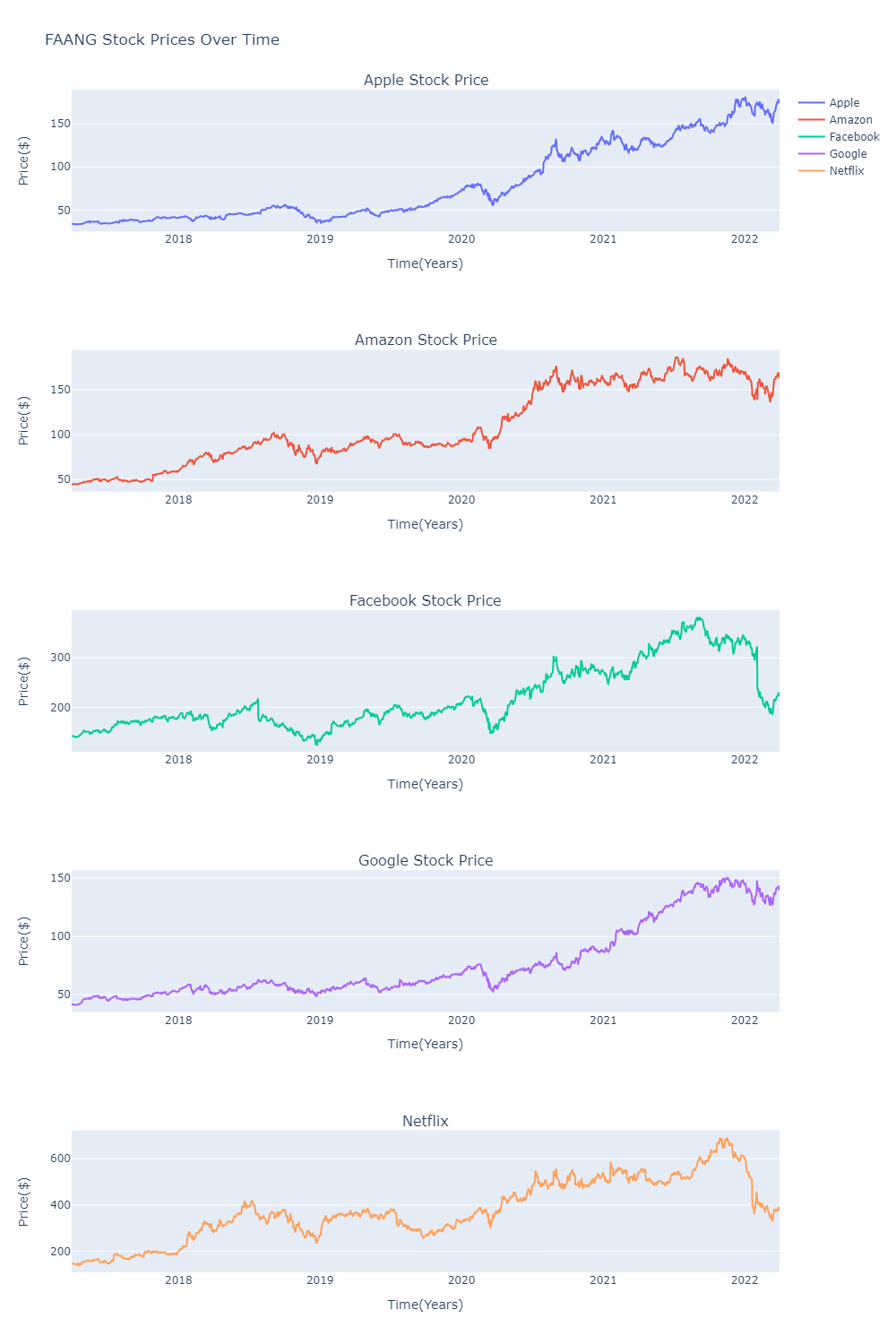Next lets calculate the returns and the risk of these stocks as well as the overall return and risk of the portfolio we have

# Calculating daily returns¶

The return of a stock is calculated as the (Ending Price – Beginning Price) / (Beginning Price)

In :
port['FB Return'] = (port['FB'] / port['FB'].shift(1)) - 1
port['AMZN Return']= (port['AMZN'] / port['AMZN'].shift(1)) - 1
port['AAPL Return'] = (port['AAPL'] / port['AAPL'].shift(1)) - 1
port['NFLX Return'] = (port['NFLX'] / port['NFLX'].shift(1)) - 1
port['GOOG Return'] = (port['GOOG'] / port['GOOG'].shift(1)) - 1
port[1:].head() #Created a new column showing daily returns of each stock

Out:
AAPL AMZN FB GOOG NFLX FB Return AMZN Return AAPL Return NFLX Return GOOG Return
Date
2017-04-03 33.869179 44.575500 142.279999 41.927502 146.919998 0.001619 0.005606 0.000278 -0.006021 0.010837
2017-04-04 34.121380 45.341499 141.729996 41.728500 145.500000 -0.003866 0.017184 0.007446 -0.009665 -0.004746
2017-04-05 33.944611 45.464001 141.850006 41.570499 143.619995 0.000847 0.002702 -0.005181 -0.012921 -0.003786
2017-04-06 33.859760 44.914001 141.169998 41.394001 143.740005 -0.004794 -0.012097 -0.002500 0.000836 -0.004246
2017-04-07 33.784340 44.743999 140.779999 41.233501 143.110001 -0.002763 -0.003785 -0.002227 -0.004383 -0.003877
In :
daily_returns = port.iloc[1:, 5:].copy() # Isolated the daily returns of our stock and stored it in a table
daily_returns.describe()

Out:
FB Return AMZN Return AAPL Return NFLX Return GOOG Return
count 1260.000000 1260.000000 1260.000000 1260.000000 1260.000000
mean 0.000635 0.001228 0.001490 0.001068 0.001125
std 0.022886 0.019616 0.019508 0.025739 0.017610
min -0.263901 -0.079221 -0.128647 -0.217905 -0.111008
25% -0.009129 -0.008075 -0.007472 -0.011554 -0.006230
50% 0.001026 0.001412 0.001176 0.000676 0.001533
75% 0.012459 0.010932 0.011510 0.014325 0.009425
max 0.108164 0.135359 0.119808 0.168543 0.104485

# Plotting the daily Returns¶

In :
# Creating subplots of the stock returns
plt.figure(figsize=(20, 40))
top_y = 0.3
low_y = -0.28

plt.subplot(5, 1, 1)
port['FB Return'].plot()
plt.ylim(low_y, top_y)
plt.title('Daily Returns for Facebook')

plt.subplot(5, 1, 2)
port['AMZN Return'].plot()
plt.ylim(low_y, top_y)
plt.title('Daily Returns for Amazon')

plt.subplot(5, 1, 3)
port['AAPL Return'].plot()
plt.ylim(low_y, top_y)
plt.title('Daily Returns for Apple')

plt.subplot(5, 1, 4)
port['NFLX Return'].plot()
plt.ylim(low_y, top_y)
plt.title('Daily Returns for Netflix')

plt.subplot(5, 1, 5)
port['GOOG Return'].plot()
plt.ylim(low_y, top_y)
plt.title('Daily Returns for Google')
plt.show()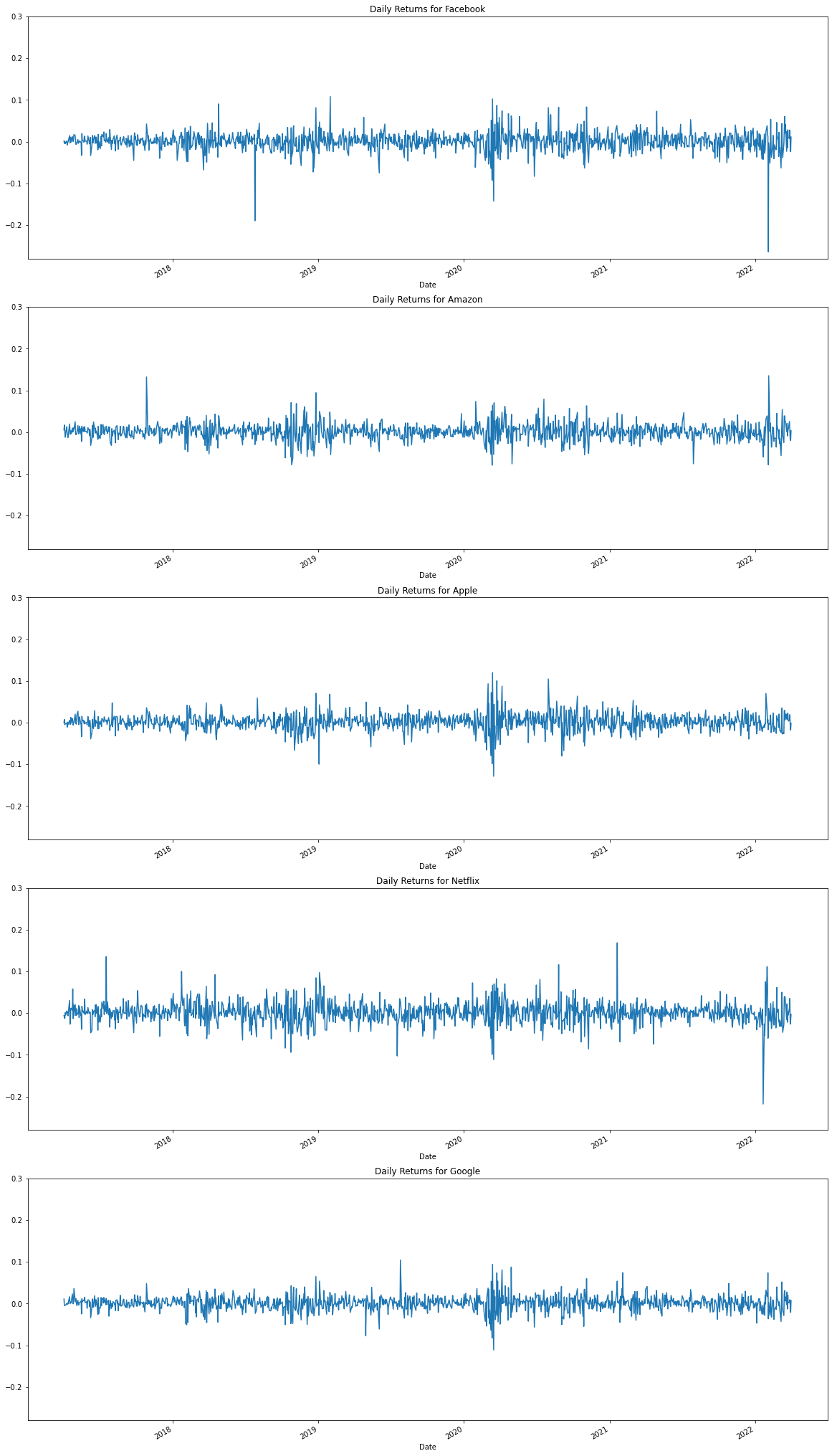On the graphs, the less variation we see in the the daily return plot overtime is indicative of the stock generating reliable returns over time. However, the more spurious the variations in the graph the less stable the returns over time are. For instance Google and Amazon seem to have generated stable returns over the observed time period, howeved in the same 5 year period, Netflix and Facebook have some huge variation points, Apple less so.

Lets now look at the annual returns of the stocks in our FAANG portfolio over the 5 year period.

## Calculating Annual Returns¶

In :
#Calculating the annual return of the portfolio
returns = (port.iloc[:, :5] / port.iloc[:, :5].shift(1)) - 1
#Assuming each security has equal weights
weights = np.array([0.20, 0.20, 0.20, 0.20, 0.20])
annual_returns = returns.mean() * 250
np.dot(annual_returns, weights)
pfolio_1 = str(round(np.dot(annual_returns, weights), 5) * 100) + ' %'
print ('The annual return of our portfolio is ' + pfolio_1)

The annual return of our portfolio is 27.731 %

In :
plt.figure(figsize=(10,5))
plt.bar(annual_returns.index, annual_returns)
plt.title('Annual Returns of FAANG Stocks')
plt.xlabel('Stock Ticker')
plt.ylabel('Annual Return')
plt.show()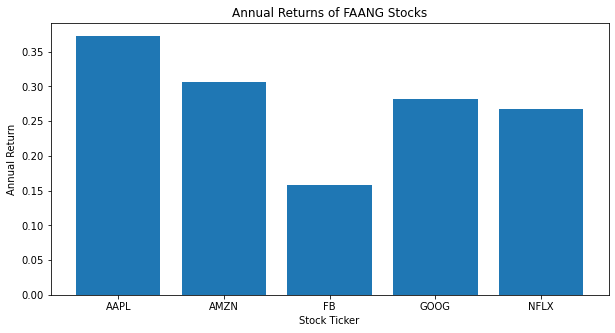Of the stock in our portfolio, AAPL seems to have generated the highest annual return, whereas FB has generated the lowest return on the same time period. AMZN has the second highest and GOOG and NFLX come third and fourth.

Lets now calculate the risk the risk profile of each stock in the portfolio.

# Calculating the volatility¶

The volatility of a stock can be measure by looking at the standard deviation of a stock. Standard deviation is defined as the deviation of the values or data from an average mean; in this instance the average mean is the return of a stock within a specific time period.

In :
daily_returns

Out:
FB Return AMZN Return AAPL Return NFLX Return GOOG Return
Date
2017-04-03 0.001619 0.005606 0.000278 -0.006021 0.010837
2017-04-04 -0.003866 0.017184 0.007446 -0.009665 -0.004746
2017-04-05 0.000847 0.002702 -0.005181 -0.012921 -0.003786
2017-04-06 -0.004794 -0.012097 -0.002500 0.000836 -0.004246
2017-04-07 -0.002763 -0.003785 -0.002227 -0.004383 -0.003877
... ... ... ... ... ...
2022-03-28 0.007979 0.025593 0.005037 0.012465 0.003028
2022-03-29 0.028042 0.001920 0.019134 0.035164 0.009158
2022-03-30 -0.008744 -0.017801 -0.006649 -0.026415 -0.004227
2022-03-31 -0.024095 -0.019865 -0.017776 -0.018036 -0.020996
2022-04-01 0.011198 0.003451 -0.001718 -0.002990 0.007522

1260 rows × 5 columns

In :
# Calculating the daily volatility of the stocks
daily_risk = returns[tickers].std()
daily_risk = (round(daily_risk, 5) * 100)
print(daily_risk.sort_values(ascending=False))

NFLX    2.574
FB      2.289
AMZN    1.962
AAPL    1.951
GOOG    1.761
dtype: float64

In :
# Calculating the annual volatility of the stocks
annual_risk = returns[tickers].std() * 250 ** 0.5
annual_risk = (round(annual_risk, 5) * 100)
print(annual_risk.sort_values(ascending=False))

NFLX    40.696
FB      36.187
AMZN    31.016
AAPL    30.845
GOOG    27.844
dtype: float64

In :
#Annual variance of the portfolio
pfolio_var = np.dot(weights.T, np.dot(daily_returns.cov() * 250, weights))

#Annual volatility of portfolio
pfolio_vol = (np.dot(weights.T, np.dot(daily_returns.cov() * 250, weights))) ** 0.5

print ('The annual variance within of our portfolio is ' + str(round(pfolio_var,5) * 100) + '%')
print ('The annual volatility of our portfolio is ' + str(round(pfolio_vol, 4) * 100) + '%')

The annual variance within of our portfolio is 7.41%
The annual volatility of our portfolio is 27.22%


# Stock Correlation and Covariance¶

Correlation in the context of the stock market describes the relationship that exists between two stocks and their respective price movements. It's important to note that correlation only measures association, but doesn't show if x causes y or vice versa—or if the association is caused by a third factor.

Covariance in the context of the stock market measures how the stock prices of two stocks (or more) move together. The two stocks prices are likely to move in the same direction if they have a positive covariance; likewise, a negative covariance indicates that they two stocks move in opposite direction.

In :
#Annual Correlation of daily returns of the stocks in our portfolio
corr_matrix = daily_returns.corr()
corr_matrix

plt.figure(figsize=(12,8))
sns.heatmap(corr_matrix, annot=True)

Out:
<AxesSubplot:>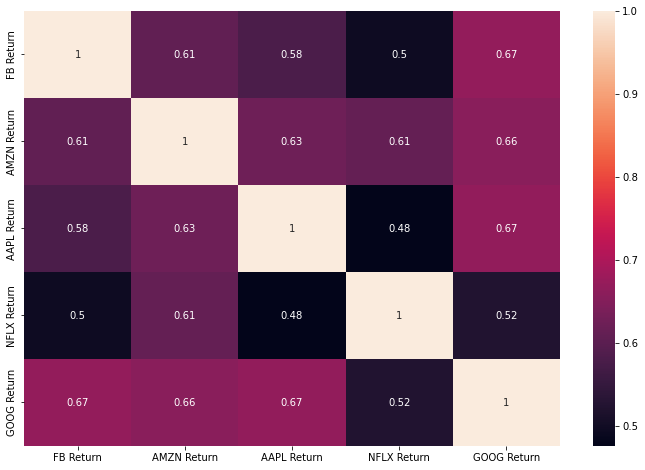Ideally, in our portfolio, we'd want our securities to have a low correlation with each other. The reason being is because stock with low correlation with each other lower the overall risk profile of a portfolio of securities. For example, if one of the stocks in our portfolio was to see a significant downturn in its return overtime, this may effect other stocks that it's has a strong correlation with. The implication could be catastrophic for your final portfolio.

One way to remove this risk is to diversify your portfolio. For example, the most common way to diversify in a portfolio of stocks is to include bonds, such as UK Gilts, as they have historically had a lower degree of correlation with the majority of stocks in financial markets.

In :
#Annual Covariance matrix of the stock in our portfolio
cov_matrix = daily_returns.cov() * 250
cov_matrix

plt.figure(figsize=(12,8))
sns.heatmap(cov_matrix, annot=True)

Out:
<AxesSubplot:>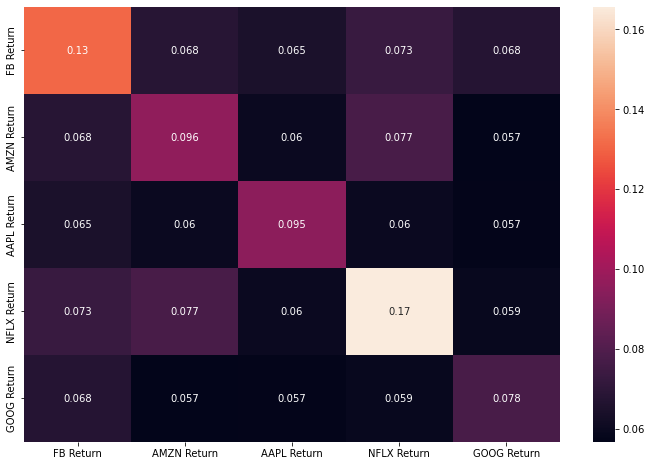Covariance is different from the correlation coefficient, a measure of the strength of a correlative relationship. Covariance is a significant tool in modern portfolio theory used to ascertain what securities to put in a portfolio. Risk and volatility can be reduced in a portfolio by pairing assets that have a negative covariance.

# Logic Behind Monte Carlo Simulations¶

How do we predict the daily return of the stock? Brownian Motion.

• Brownian motion will be the main driver for estimating the return. It is a stochastic process used for modeling random behavior over time. Brownian motion has two main components:

• Drift — the direction that rates of returns have had in the past. That is, the expected return of the stock. You may ask yourself: why is the variance multiplied by 0.5? Becasue historical values are eroded in the future.
• Volatility — the historical volatility multiplied by a random, standard normal variable.m

### Computing the logarithmic return and variance of the stock¶

In :
log_returns = np.log(1 + port['AAPL'].pct_change())
u = log_returns.mean()

In :
var = log_returns.var()


### Computing the drift of the stock¶

In :
drift = (u - (0.5 * var))

In :
stdev = log_returns.std()


### Forecast selection¶

In :
t_intervals = 250 #No. of day we want to forecast price for
iterations = 10 #No. of outcomes we want to observer


### Calculating the daily returns forecast¶

In :
from scipy.stats import norm
daily_returns_apple = np.exp(drift + stdev * norm.ppf(np.random.rand(t_intervals, iterations)))
daily_returns_apple

Out:
array([[0.99683947, 1.01280178, 0.96979297, ..., 1.02620878, 0.98586883,
0.98301438],
[0.99225112, 1.00515811, 0.98920124, ..., 1.0410217 , 1.00392954,
1.01725459],
[1.011996  , 1.00559282, 0.96770158, ..., 0.99461928, 1.02444441,
0.97403408],
...,
[0.99027911, 0.97552546, 1.0196474 , ..., 0.97297744, 0.99976208,
1.01657839],
[0.98358822, 0.9712289 , 1.0065741 , ..., 1.02030409, 0.99858461,
0.98341568],
[0.96324573, 1.03948864, 0.98313166, ..., 1.01027925, 0.98408428,
1.00913343]])
In :
daily_returns_apple.shape

Out:
(250, 10)

### Forecasting stock prices¶

In :
S0 = port['AAPL'].iloc[-1]
S0

Out:
174.05426025390625
In :
price_list = np.zeros_like(daily_returns_apple)
price_list # Create a variable price_list with the same dimension as the daily_returns matrix

Out:
array([[0., 0., 0., ..., 0., 0., 0.],
[0., 0., 0., ..., 0., 0., 0.],
[0., 0., 0., ..., 0., 0., 0.],
...,
[0., 0., 0., ..., 0., 0., 0.],
[0., 0., 0., ..., 0., 0., 0.],
[0., 0., 0., ..., 0., 0., 0.]])
In :
price_list.shape

Out:
(250, 10)
In :
price_list

Out:
array([0., 0., 0., 0., 0., 0., 0., 0., 0., 0.])
In :
price_list = S0
price_list #Set the values on the first row of the price_list array equal to S0

Out:
array([[174.05426025, 174.05426025, 174.05426025, ..., 174.05426025,
174.05426025, 174.05426025],
[  0.        ,   0.        ,   0.        , ...,   0.        ,
0.        ,   0.        ],
[  0.        ,   0.        ,   0.        , ...,   0.        ,
0.        ,   0.        ],
...,
[  0.        ,   0.        ,   0.        , ...,   0.        ,
0.        ,   0.        ],
[  0.        ,   0.        ,   0.        , ...,   0.        ,
0.        ,   0.        ],
[  0.        ,   0.        ,   0.        , ...,   0.        ,
0.        ,   0.        ]])
In :
for t in range(1, t_intervals):
price_list[t] = price_list[t - 1] * daily_returns_apple[t]

In :
price_list

Out:
array([[174.05426025, 174.05426025, 174.05426025, ..., 174.05426025,
174.05426025, 174.05426025],
[172.7055344 , 174.95205211, 172.17468925, ..., 181.19426187,
174.73821381, 177.0574954 ],
[174.77731048, 175.9305283 , 166.61371947, ..., 180.21930672,
179.0095859 , 172.46003484],
...,
[206.79150989, 444.43768941, 218.49071748, ..., 161.59426382,
341.51229758, 230.070622  ],
[203.39769306, 431.65072929, 219.92709732, ..., 164.8752886 ,
341.02892419, 226.25505664],
[195.92196032, 448.69603168, 216.21729151, ..., 166.57008227,
335.60120368, 228.32154167]])
In :
plt.figure(figsize=(15,6)) #Plotting the price forecast we made using the simulation
plt.title('1 Year Monte Carlo Simulation for Apple')
plt.ylabel("Price ($)") plt.xlabel("Time (Days)") plt.plot(price_list) plt.show()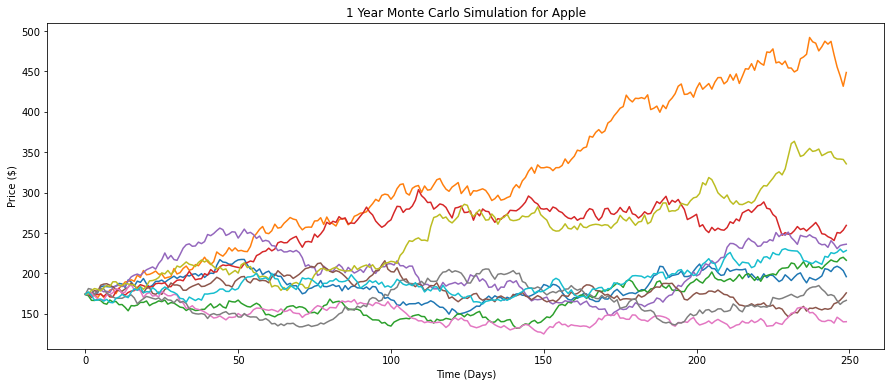## Extending Prediction Visualisation¶ In : import plotly.express as px price_list = pd.DataFrame(price_list) price_list = price_list.set_axis(['Forecast 1', 'Forecast 2', 'Forecast 3', 'Forecast 4', 'Forecast 5', 'Forecast 6', 'Forecast 7', 'Forecast 8', 'Forecast 9', 'Forecast 10'], axis=1, inplace=False) fig = px.line(data_frame=price_list, x=price_list.index, y=price_list.columns, labels={'value': 'Price($)',
'index': 'Time (Days)',
'variable':'Simulations '},
title='1 Year Monte Carlo Simulation for Apple'
)
fig.update_layout(height=500, width=1000)
fig.show('png')